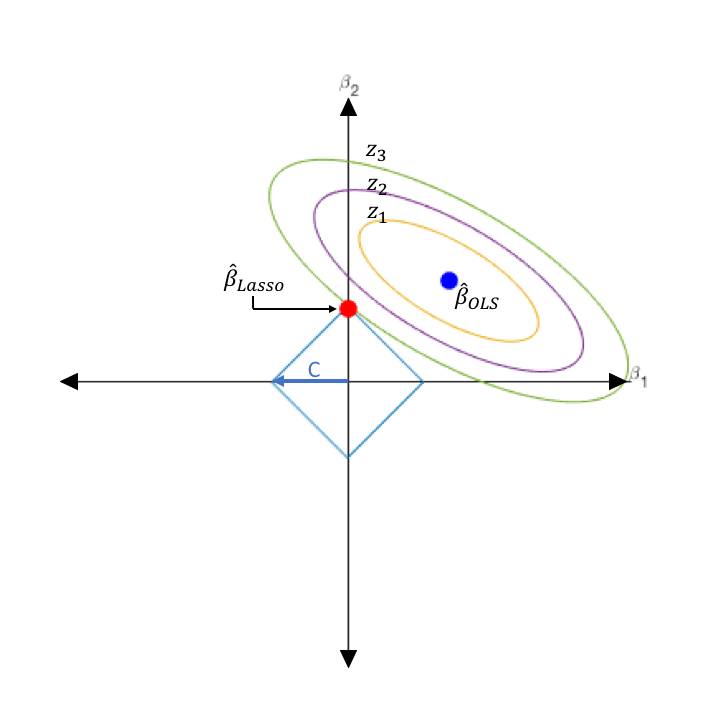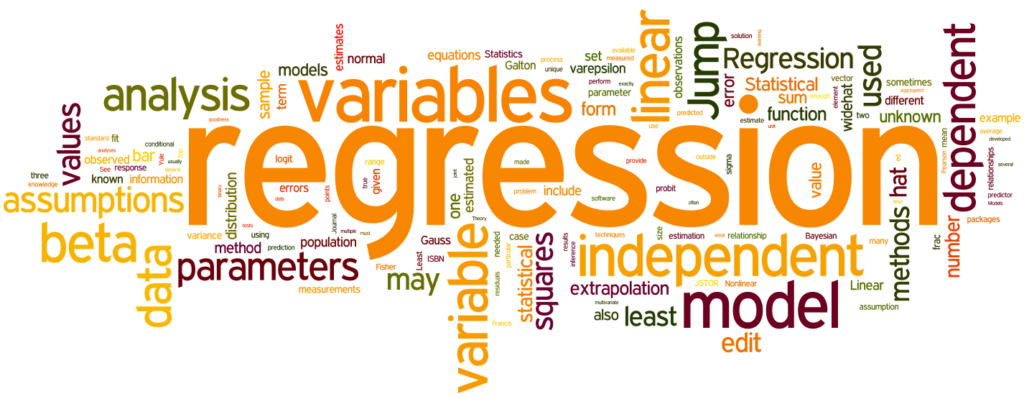In this blog post, I will first try to explain the basics of Lasso Regression. Then, we’ll build the model using a dataset with Python. Finally, we’ll evaluate the model by calculating the mean square error. Let’s get started step by step.Resource: https://waterprogramming.wordpress.com/2017/02/22/dealing-with-multicollinearity-a-brief-overview-and-introduction-to-tolerant-methods/

### What is the Lasso Regression?

The main purpose in Lasso Regression is to find the coefficients that minimize the error sum of squares by applying a penalty to these coefficients. In another source, it is defined as follows:

The “LASSO” stands for Least Absolute Shrinkage and Selection Operator. Lasso regression is a regularization technique. It is used over regression methods for a more accurate prediction. This model uses shrinkage. Shrinkage is where data values are shrunk towards a central point as the mean. Lasso Regression uses L1 regularization technique. It is used when we have more number of features because it automatically performs feature selection.

#### Features of Lasso Regression

• Ridge Regression’s all relevant-unrelated variables have been proposed to overcome the disadvantage of leaving the model.
• Lasso Regression brings the coefficients closer to zero.
• But when the norm L1 is large enough, it makes some coefficients zero. Thus, the variable makes the selection.
• It is very important that λ is chosen correctly. Cross-Validation is used for this.
• Ridge and Lasso methods are not superior to each other.Resource: https://spotio.com/blog/regression-analysis/

### Lasso Regression Model

• λ denotes the amount of shrinkage.
• λ = 0 implies all features are considered and it is equivalent to the linear regression where only the residual sum of squares is considered to build a predictive model
• λ = ∞ implies no feature is considered i.e, as λ closes to infinity it eliminates more and more features
• The bias increases with an increase in λ
• Variance increases with a decrease in λ

### Modeling with Python

Now let’s build a Lasso Regression model on a sample data set. And then let’s calculate the square root of the model’s Mean Squared Error. This will give us the model error.

First of all, we import the libraries necessary for modeling as usual.